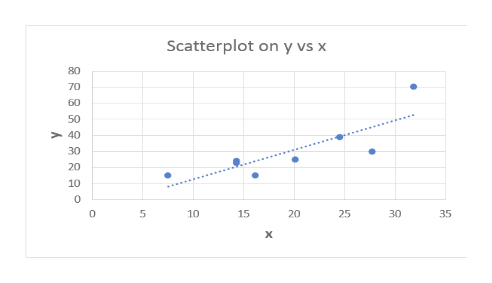# Do larger universities tend to have more property crime? University crime statistics are affected by a variety of factors. The surrounding community, accessibility given to outside visitors, and many other factors influence crime rate. Let x be a variable that represents student enrollment (in thousands) on a university campus, and let y be a variable that represents the number of burglaries in a year on the university campus. A random sample of n = 8 universities in California gave the following information about enrollments and annual burglary incidents.x14.331.824.514.37.527.716.220.1y2470392315301525(a) Make a scatter diagram of the data. Flash Player version 10 or higher is required for this question. You can get Flash Player free from Adobe's website. Then visualize the line you think best fits the data. (b) Use a calculator to verify that Σx = 156.4, Σx2 = 3510.46, Σy = 241, Σy2 = 9501 and Σxy = 5542.6.Compute r. (Round to 3 decimal places.) As x increases, does the value of r imply that y should tend to increase or decrease? Explain your answer.Given our value of r, y should tend to decrease as x increases.Given our value of r, y should tend to remain constant as x increases. Given our value of r, y should tend to increase as x increases.Given our value of r, we can not draw any conclusions for the behavior of y as x increases.

Question
261 views

Do larger universities tend to have more property crime? University crime statistics are affected by a variety of factors. The surrounding community, accessibility given to outside visitors, and many other factors influence crime rate. Let x be a variable that represents student enrollment (in thousands) on a university campus, and let y be a variable that represents the number of burglaries in a year on the university campus. A random sample of n = 8 universities in California gave the following information about enrollments and annual burglary incidents.

 x 14.3 31.8 24.5 14.3 7.5 27.7 16.2 20.1 y 24 70 39 23 15 30 15 25
(a) Make a scatter diagram of the data.

Flash Player version 10 or higher is required for this question.
You can get Flash Player free from Adobe's website.

Then visualize the line you think best fits the data.

(b) Use a calculator to verify that Σx = 156.4, Σx2 = 3510.46, Σy = 241, Σy2 = 9501 and Σxy = 5542.6.

Compute r. (Round to 3 decimal places.)

As x increases, does the value of r imply that y should tend to increase or decrease? Explain your answer.
Given our value of ry should tend to decrease as x increases.
Given our value of ry should tend to remain constant as x increases.
Given our value of ry should tend to increase as x increases.
Given our value of r, we can not draw any conclusions for the behavior of y as x increases.
check_circle

Step 1

(a) Scatter diagram of the data:

The least-squares regression line is the best fitted to the given data.

The procedure to draw scatterplot with least-squares line using EXCEL is as follows.

• Select the data x and y.
• Go to Insert.
• Select Scatter under Charts.
• Under chart elements, check Trendline.

The scatter diagram for the data that is obtained using EXCEL is as followshelp_outlineImage TranscriptioncloseScatterplot on y vs x 80 70 60 50 40 30 20 10 C 10 15 20 25 30 35 X fullscreen
Step 2

(b) Computation of r:

Using calculator, the following preliminary calculations verified.

∑x = (14.3 + 31.8 + 24.5 + 14.3 + 7.5 + 27.7 + 16.2 + 20.1) = 156.4.

∑x2 = (14.32 + 31.82 + 24.52 + 14.32 + 7.52 + 27.72 + 16.22 + 20.12) = 3,510.46.

∑y = (24 + 70 + 39 + 23 + 15 + 30 + 15 + 25) = 241.

∑y2 = (242 + 702 + 392 + 232 + 152 + 302 + 152 + 252) = 9,501.

∑xy = (14.3×24) + (31.8×70) + (24.5×39) + (14.3×23) + (7.5×15) + (27.7×30) + (16.2×15)  + (20.1×25) = 5,542.6.

Here, the number of pair of observations, n = 8.

The EXCEL formula to find the value of correlation coefficient, r is “=CORREL(array1, array2)”.

The correlation coefficient, r is calculated as 0.825 using the EXCEL formula “=CORREL(A3:A10,B3:B10)”.

Step 3

Interpretation of correlation coefficient:

The correlation coefficient is 0.825 which is a positive value. This represents that as student enrolment on a university campus (x) increases, the number of bur...

### Want to see the full answer?

See Solution

#### Want to see this answer and more?

Solutions are written by subject experts who are available 24/7. Questions are typically answered within 1 hour.*

See Solution
*Response times may vary by subject and question.
Tagged in

### Other